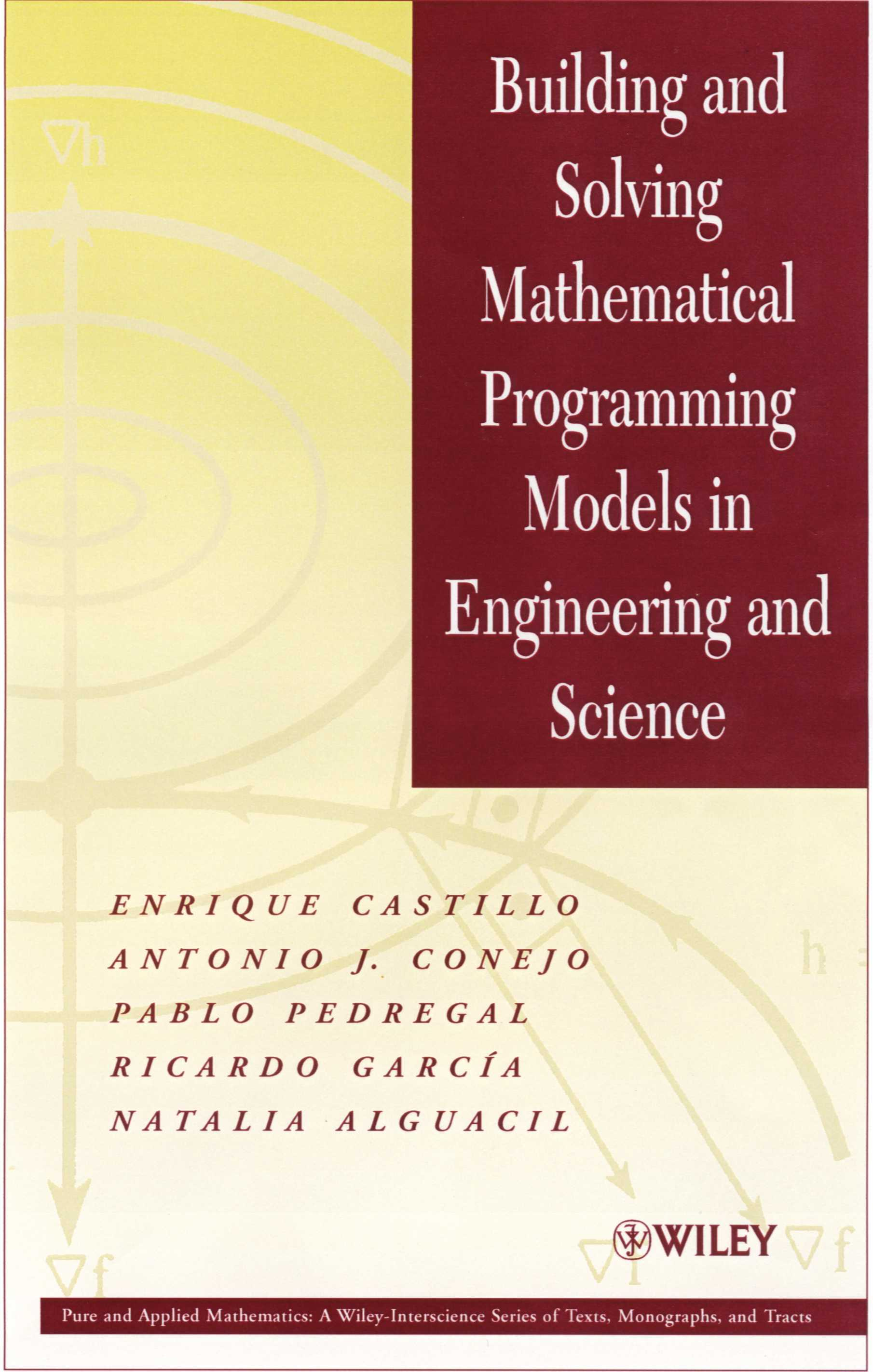Building and Solving Mathematical Programming Models in Engineering and Science

(This material is used by permission of John Wiley and Sons, Inc.)

#Castillo, E., Conejo A.J., Pedregal, P., García, R. and Alguacil, N. (2002) "Building and Solving Mathematical Programming Models in Engineering and Science", Pure and Applied Mathematics Series, Wiley, New York.

The book is organized in four parts. In Part I we deal with models to introduce the reader to the attractive field of mathematical programming by means of carefully selected examples. We guide the reader to discover the mathematical statement of the problems after a clear understanding of the physics or engineering problems.

Part II deals with methods and describes the main techniques used to solve linear and nonlinear programming problems. As indicated above, the aim of this part is to give not a rigorous analysis of the methods but a clear understanding of the main ideas.

Since nothing can be practically done without the help of good software, in Part III we have selected GAMS (the general algebraic modeling system) as the main tool to be used in the book. To facilitate its use, a detailed description of how problems need to be stated and the possibilities of GAMS are given. All the problems in Part I and many others are solved using this tool. The current version of these programs, together with a free demo version and a brief User's Guide, can be obtained from the World Wide Web site http://www.gams.com/

Once the reader is familiar with mathematical programming problems and the GAMS software, Part IV is devoted to more dense applications of these techniques to practical problems in many areas of research, including artificial intelligence (AI), computer assisted design (CAD), probability and statistics, economics, engineering, and transportation. All these applications have arisen from real life. In other words, they are mathematical models of physical, economic, or engineering problems. This part also includes a chapter dedicated to some tricks that are useful in dealing with especial cases of problems as, for example, converting some nonlinear problems into linear ones, and it also gives some specific GAMS tricks.

This book can be used as a reference or consulting book and as a textbook in upper-division undergraduate courses or in graduate-level courses. Included in the book are numerous illustrative examples and end-of-chapter exercises. We have also developed the GAMS code to implement the various examples and methodologies presented in this book.

This book is addressed to a wide audience, including mathematicians, engineers, and applied scientists.